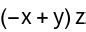GROUP THEORY TUTORIAL

# Representation matrices and projection operators

During this tutorial we are going to show how to obtain representation matrices of irreducible representations and how to apply projection operators based on the irreducible representations.

 GTGetIreps gives the character table and representation matrices of irreducible representations GTCharProjectionOperator applies the character projection operator GTProjectionOperator gives the part of a given function which transforms like the m-th row and the n-th column of an irreducible representation
For our example we consider the point group D4h. We install D4h using GTInstallGroup. The character Table and the representation matrices are obtained using GTGetIreps.
 In:=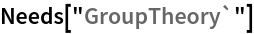In:=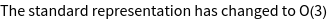Out=In:=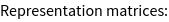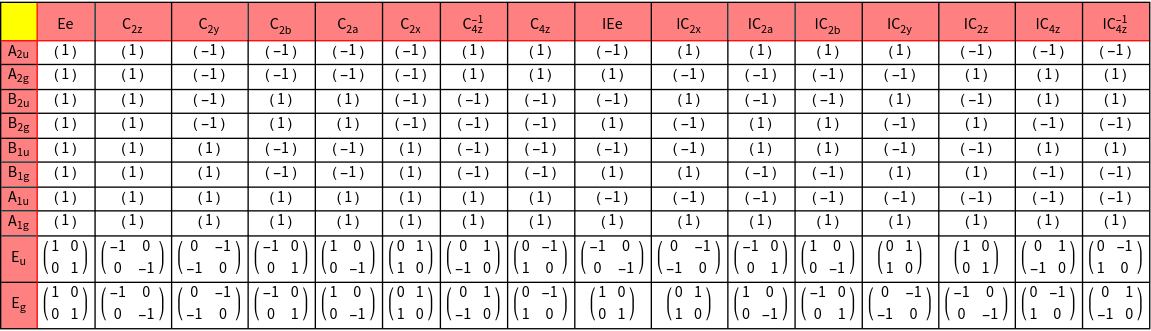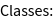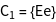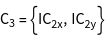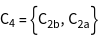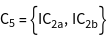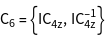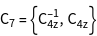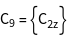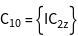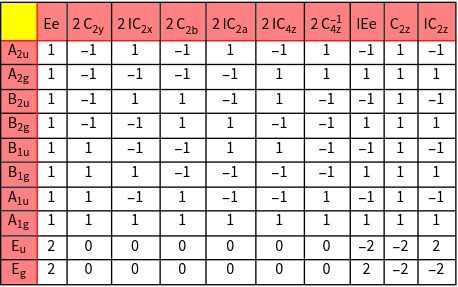We choose the irreducible representation B1g as well as the irreducible representation Eg.
 In:=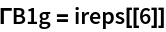Out=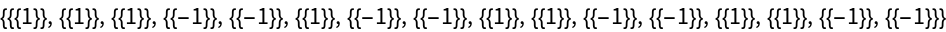In:=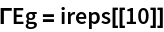Out=To apply projection operators, we need to specify a function, which we choose to be f(x, y, z) = x2y2 + x y + x z + z y. The character projection operator is applied using GTCharProjectionOperator. Here we can either choose a specific irreducible representation by providing its characters, or it can be applied for all irreducible representations by providing the full character system. As can be verified, the part x y transforms as B2g, x2-y2 transforms as B1g, and x z + y z transforms as Eg.
 In:=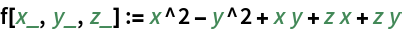In:=Out=In:=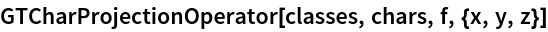Out=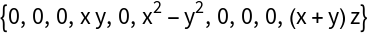Eg is a two-dimensional representation and hence we can find two linearly independent basis functions spanning the basis of this representation. By applying the full projection operator GTProjectionOperator for the different diagonal components of the representation matrices, we can project out two such functions.
 In:=Out=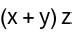In:=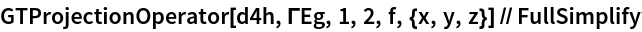Out=